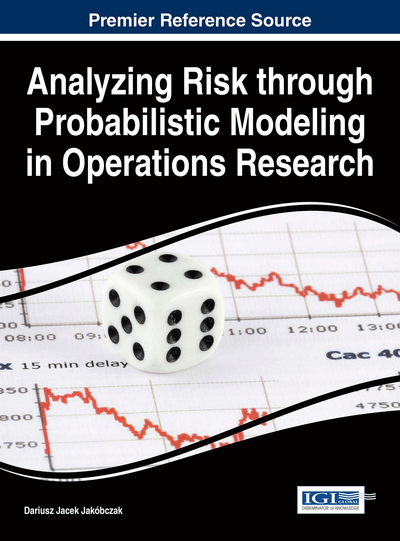# Simulation Output Analysis and Risk Management

E. Jack Chen (BASF Corporation, USA)
DOI: 10.4018/978-1-4666-9458-3.ch009

## Abstract

Computer simulation is the process of designing and creating a computerized model of a real or proposed system for the purpose of conducting experiments to give us a better understanding of the behavior of the system under study for a given set of condition. Simulation studies have been used to investigate the characteristics of systems, to assess and analyze risks, for example, the probability of a machine breakdown. Hence, simulation is a valuable tool for risk management. However, estimates of measure of system performance from stochastic simulation are themselves random variables and are subject to sampling error. One must take into account sampling error when making inferences concerning system performance. We discuss how statistical techniques are applied in simulation output analysis, e.g., initialization bias reduction, tests of independence, confidence interval estimation, and quantile estimation. A carefully selected quantiles can reveals characteristic of the underlying distribution. These statistical techniques are key components of many simulation studies.
Chapter Preview
Top

## Introduction

Computer simulation refers to methods and applications for studying a variety of models of real-world systems by numerical evaluation using software developed to mimic the behavior of the systems. Simulation output usually consists of one or more random variables because of the stochastic nature of the output data and output analysis refers to the examination of the data generated by a simulation. Simulation studies have been used to investigate the characteristics of systems, to assess and analyze risks, for example, the probability of a machine breakdown. For general reference of computer simulation, see Law (2014).

In many applications we want to estimate the probability that a future observation will exceed a given level during some specified epoch. For example, a machine may break down if a certain temperature is reached. Among many other aspects, reliability analysis (risk analysis) studies the expected life and the failure rate of a component or a system of components linked together in some structure. It is often of interest to estimate the reliability of the component/system from the observed lifetime data. With probabilistic modeling, normal and lognormal densities are commonly used to model certain lifetimes in reliability and survival analysis as well as risk management.

Risk management is the identification, assessment, and prioritization of risks to minimize the probability of unfortunate events, e.g., machine break down, loss of financial assets, cyberattacks. Inadequate risk management can result in severe consequences for individuals as well as the entire society. For instance, the U.S. subprime mortgage crisis and the associated recession were largely caused by the loose credit risk management of financial firms. Risk management often starts with a probabilistic risk assessment, a systematic and comprehensive methodology to identify and evaluate risks. Based on the assessment, the planner then comes up a strategy and/or plan to minimize the loss (cost), or to maximize the return (opportunity). The simplest models (of analyzing risks) often consist of a probability multiplied by an impact (severity of the possible adverse consequences). Understanding risks may be difficult as multiple factors (impacts) can contribute to the total probability of risk. Consider a device contains a series of two identical components and the lifetime of the component, where “” denotes “is distributed as” and “” denotes a uniform distribution with range [a,b]. If any of these two components fail, the device fails, such as chains. Then, the lifetime of the device. On the other hand, if these two components are configured in parallel and the device fails only when both components fail, such as a strand contains a bundle of threads, then the lifetime of the device. However, nearly all systems consist of many components and processes, the interactions of those components and processes make the analysis complicated if not impossible. Hence, analytical solution may not be feasible.

## Complete Chapter List

Search this Book:
Reset